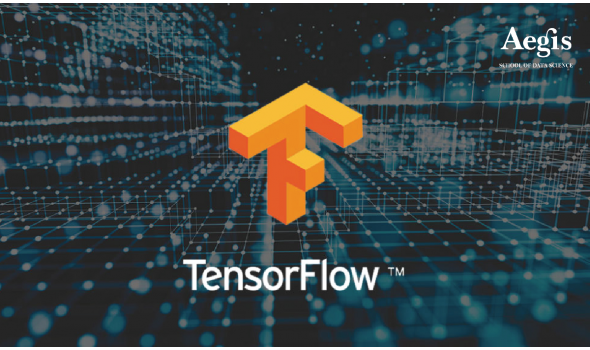#### Deep Learning with Tensorflow#### Details

Certification Body: Aegis School of Data Science On-campus,Online Certificate course English 25000 INR Application ★★★★★No Ratings

# Course Details

#### Description:

TensorFlow is an open source software library for numerical computation using data flow graphs. Nodes in the graph represent mathematical operations, while the graph edges represent the multidimensional data arrays (tensors) communicated between them. The flexible architecture allows you to deploy computation to one or more CPUs or GPUs in a desktop, server, or mobile device with a single API. TensorFlow was originally developed by researchers and engineers working on the Google Brain Team within Google's Machine Intelligence research organization for the purposes of conducting machine learning and deep neural networks research, but the system is general enough to be applicable in a wide variety of other domains as well. TensorFlow is comprised of numerical computation using data flow graphs, wherein nodes represent mathematical operations, while the graph edges represent the multidimensional data arrays (tensors) communicated between them. The flexible architecture of tensorflow makes the deployment of the machine learning and deep learning models easier in a desktop, server, or mobile device.

This Deep Learning with TensorFlow course focuses on TensorFlow. Traditional neural networks rely on shallow nets, composed of one input, one hidden layer and one output layer. Deep-learning networks are distinguished from these ordinary neural networks having more hidden layers, or so-called more depth. These kind of nets are capable of discovering hidden structures within unlabeled and unstructured data (i.e. images, sound, and text), which consitutes the vast majority of data in the world. TensorFlow is one of the best libraries to implement deep learning. TensorFlow is a software library for numerical computation of mathematical expressional, using data flow graphs. Nodes in the graph represent mathematical operations, while the edges represent the multidimensional data arrays (tensors) that flow between them. It was created by Google and tailored for Machine Learning. In fact, it is being widely used to develop solutions with Deep Learning. In this TensorFlow course, you will be able to learn the basic concepts of TensorFlow, the main functions, operations and the execution pipeline. Starting with a simple “Hello Word” example, throughout the course you will be able to see how TensorFlow can be used in curve fitting, regression, classification and minimization of error functions. This concept is then explored in the Deep Learning world. You will learn how to apply TensorFlow for backpropagation to tune the weights and biases while the Neural Networks are being trained. Finally, the course covers different types of Deep Architectures, such as Convolutional Networks, Recurrent Networks and Autoencoders.

This course will guide you through how to use Google's TensorFlow framework to create artificial neural networks for deep learning! This course aims to give you an easy to understand guide to the complexities of Google's TensorFlow framework in a way that is easy to understand. Other courses and tutorials have tended to stay away from pure tensorflow and instead use abstractions that give the user less control. Here we present a course that finally serves as a complete guide to using the TensorFlow framework as intended, while showing you the latest techniques available in deep learning!

This course is designed to balance theory and practical implementation, with complete jupyter notebook guides of code and easy to reference slides and notes. We also have plenty of exercises to test your new skills along the way!

#### Course details:

Pre-requisites:

• Machine Learning (Intermediate level)
• Python programming (Beginner level)
• Basic knowledge of math and statistics (mean, standard deviation, etc..)

Hardware and OS requirements:

• 40 GB free disk space
• 4 GB RAM (8 GB preferred)
• Any recent Intel processor
• Any recent NVIDIA GPU (preferred)
• Windows 7 (64-bit) Operating System or later
• High speed Internet connectivity

Software requirement: Any browser application IE or Chrome or Firefox

Objective: To help participants, for solving real world problems by using Deep Learning concepts

Course Curriculum:

• Introduction
• Installation and Setup
• What is Machine Learning?
• Crash Course Overview
• Introduction to Neural Networks
• TensorFlow Basics
• Convolutional Neural Networks
• Recurrent Neural Networks
• AutoEncoders
• Reinforcement Learning with Tensorflow and OpenAI Gym
• GAN - Generative Adversarial Networks